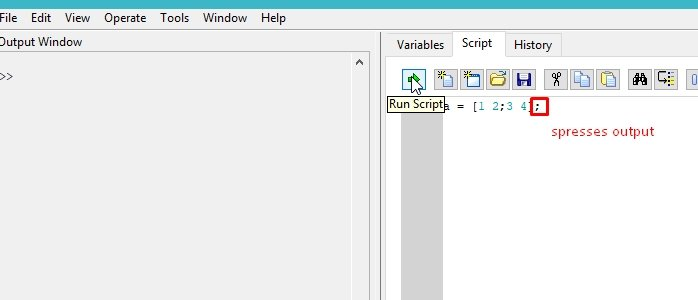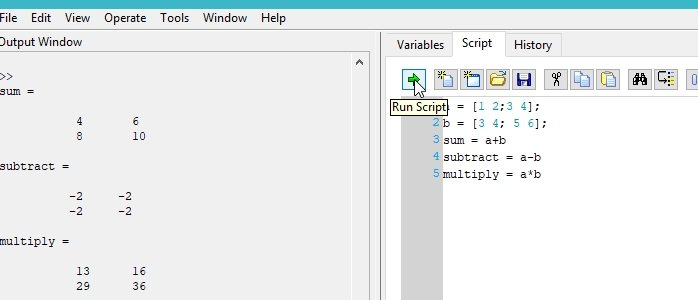# Matrix Addition subtraction and multiplication in labview : tutorial 29

In this tutorial I will help you to deal with matrices in LabView. LabView has provided us with an option of MathSript in which you can write you code in mathscript and run it as simple LabView code. If you want to manipulate matrices I will suggest you to use mathscript window as we have used before for basic introduction of mathscript window, because it is way easier than 2D arrays manipulation in block diagram designing. In this tutorial you will leanr hoe to Add subtract, and multiply two matrices in LabView with the help of a mathscript code. At the end of the tutorial you are provided with an exercise to do it by yourself, and in the next tutorials I will assume that you have done those exercises and I will not explain the concept regarding them. See our tutorial on using mathscripts in labview

### Matrix Addition subtraction and multiplication in labview

Figure 1: Opening mathscript

Figure 2: Opening script

Figure 3: Saving script

Figure 4: Matrix definition

Figure 5: Running a script

Figure 6: Matrix output

• This implies that separating two arrays by a semicolon (;) will generate a matrix with the array before the semicolon as first row of the matrix and array after the semicolon as second row of the matrix as can be seen from the output window.
• If you don’t want each line of your code to be displayed on the output window, simply place a semicolon at the end of the line, this semicolon will suppress the output of the command to be displayed on the output window.
• By default every mathscript command line has a printing option with it and the semicolon will tell LabView to suppress the printing option of the command as shown in the figure below,Figure 7: Suppressing the output

Figure 9: Matrix subtraction

• Coming over to the matrix multiplication part, we all know that matrix multiplication is not as straight forward as simple addition and subtraction case. In order to evaluate matrix multiplication we have to take into account the rules defined to multiply two matrices.
• One of all the major benefits of using mathscript is that we can evaluate matrix multiplication by simply placing an asterisk (*) sign between two matrices. Placing a ‘*’ sign between two matrices will multiply one matrix with the other matrices according to matrix rules of multiplication as shown in the figure below,Figure 10: Matrix multiplication

Figure 11: Element wise multiplication

Exercise:

• Do all the tasks we have done in this tutorial using a matrix of sixe 3×3.

<< Previous tutorial                                                    Next tutorial>>